# Options¶

Learn how to create options for pickers. Options can be created from strings, sequences, tuples, or dictionaries.

## Basic¶

An `option` represents one of several choices to be presented to the user. It's used by all kinds of pickers: buttons, dropdowns, checklists, color pickers, and so on.

An option has a `value` and `text`, created using `option(value, text)`.

• The `value` is the value returned when the user picks that option. It is not user-visible.
• The `text` is typically used as a label for the option.

If `text` is not provided, then the `value` is also used as the `text`.

There are other, more concise ways to specify options, explained in later examples.

``````choice = view(box('Choose a color', options=[
option('green', 'Green'),
option('yellow', 'Yellow'),
option('orange', 'Orange'),
option('red', 'Red'),
]))
view(f'You chose {choice}.')
``````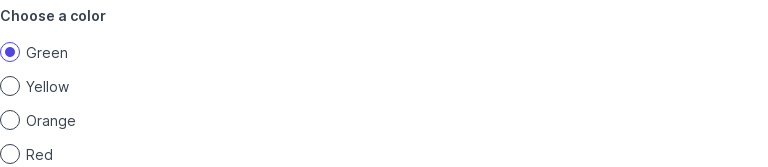## Create options from a sequence¶

If `options` is a sequence (tuple, set or list), the elements of the sequence are used as options.

``````choice = view(box('Choose a color', options=[
'green', 'yellow', 'orange', 'red'
]))
view(f'You chose {choice}.')
``````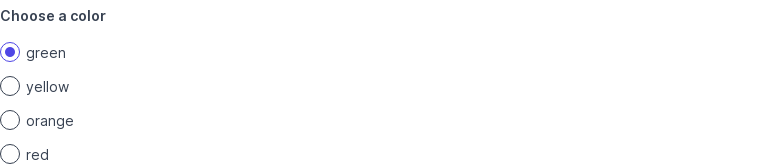## Create options from a string¶

If `options=` is set to a string, each word in the string is used as an option.

``````choice = view(box('Choose a color', options='green yellow orange red'))
view(f'You chose {choice}.')
``````

In other words, `'green yellow orange red'` is shorthand for `['green', 'yellow', 'orange', 'red']`.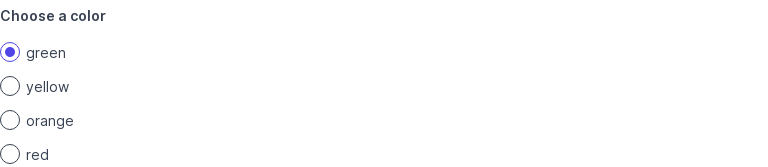## Create options from tuples¶

`options=` can also be specified as a sequence of `(value, text)` tuples.

``````choice = view(box('Choose a color', options=[
('green', 'Green'),
('yellow', 'Yellow'),
('orange', 'Orange'),
('red', 'Red'),
]))
view(f'You chose {choice}.')
``````

Here, `(value, text)` is shorthand for `option(value, text)`.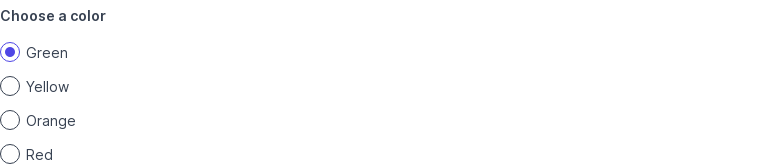## Create options from a dictionary¶

`options=` can also be specified as a `dict` of `value: text` entries.

``````choice = view(box('Choose a color', options=dict(
green='Green',
yellow='Yellow',
orange='Orange',
red='Red',
)))
view(f'You chose {choice}.')
``````

This is the most concise way to pass options where labels differ from values.## Mark options as selected¶

Set `selected=True` to pre-select an option.

Another way to pre-select an option is to set `value=` on the box, as shown in the next example.

``````choice = view(box('Choose a color', options=[
option('green', 'Green'),
option('yellow', 'Yellow', selected=True),
option('orange', 'Orange'),
option('red', 'Red'),
]))
view(f'You chose {choice}.')
``````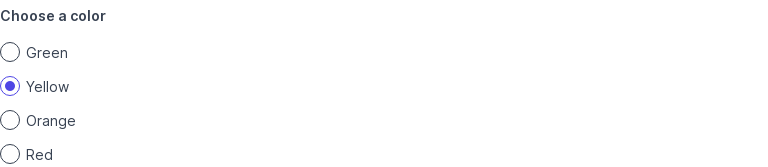## Set initial selection¶

Set `value=` on the box to pre-select an option having that value.

Another way to pre-select an option is to set `selected=True` on the option, as shown in the previous example.

``````choice = view(box('Choose a color', value='yellow', options=[
option('green', 'Green'),
option('yellow', 'Yellow'),
option('orange', 'Orange'),
option('red', 'Red'),
]))
view(f'You chose {choice}.')
``````